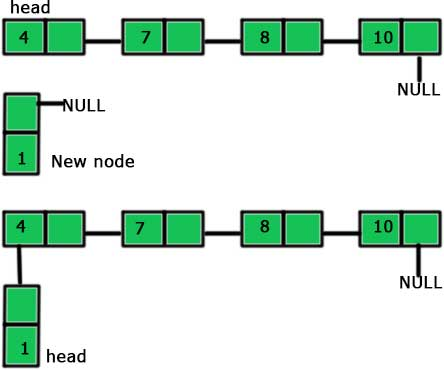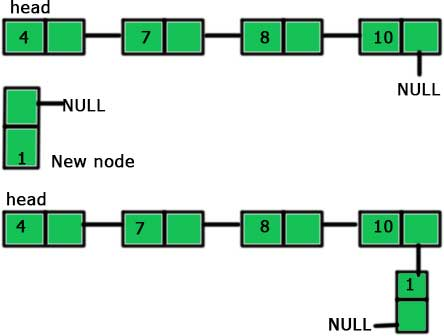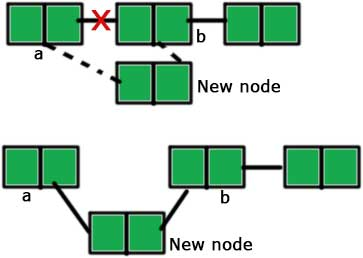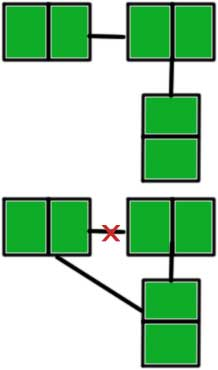# Inserting a new node in a linked list in C.

May 25, 2017 234288

### Previous:

Make sure that you are familiar with the concepts explained in the article(s) mentioned above before proceeding further.

We will proceed further by taking the linked list we made in the previous article.

#include <stdio.h>
#include <stdlib.h>

struct node
{
int data;
struct node *next;
};

int main()
{
int n,i;
printf ("number of elements:");
scanf("%d",&n);
for(i=0;i<n;i++)
{
p=malloc(sizeof(struct node));
scanf("%d",&p->data);
p->next=NULL;
else
prev->next=p;
prev=p;
}
return 0;
}


There are three different possibilities for inserting a node into a linked list. These three possibilities are:

1. Insertion at the beginning of the list.
2. Insertion at the end of the list
3. Inserting a new node except the above-mentioned positions.

In the first case, we make a new node and points its next to the head of the existing list and then change the head to the newly added node. It is similar to picture given below.So, the steps to be followed are as follows:

1. Make a new node
2. Point the ‘next’ of the new node to the ‘head’ of the linked list.
3. Mark new node as ‘head’.

Thus, the code representing the above steps is:

struct node* front(struct node *head,int value)
{
struct node *p;
p=malloc(sizeof(struct node));
p->data=value;
return (p);
}

/*
main funtion will be something like:
main()
{
}
*/


The code is very simple to understand. We just made a new node in these three lines of the code:

struct node *p; p=malloc(sizeof(struct node)); p->data=value;

p->next=head – In this line, we have followed the second step which is to point the ‘next’ of the new node to the head of the linked list.

return (p); head=front(head,10);

These two lines are the part of marking the new node as ‘head’. We are returning the new node from our function and making it head in the main function.

The second case is the simplest one. We just add a new node at the end of the existing list. It is shown in the picture given below:So, the steps to add the end if a linked list are:

1. Make a new node
2. Point the last node of the linked list to the new node

And the code representing the above steps are:

end(struct node *head,int value)
{
struct node *p,*q;
p=malloc(sizeof(struct node));
p->data=value;
p->next=NULL;
while(q->next!=NULL)
{
q = q->next;
}
q->next = p;
}
/*
main function will contain something like:
*/


p=malloc(sizeof(struct node)); p->data=value; p->next=NULL;

The above-mentioned lines are just creating a new node.

while(q->next!=NULL) {  q = q->next; }

We are traversing to the end of the list using the above lines of code to make ‘q’ the last element of the list.

Now ‘q’  is the last element of the list, so we can add the new node next to it and we are doing the same by the code written after the while loop:

q = q->next

The third and the last case is a little bit complicated. To insert a node in between a linked list, we need to first break the existing link and then create two new links. It will be clear from the picture given below.The steps for inserting a node after node ‘a’ (as shown in the picture) are:

1. Make a new node
2. Point the ‘next’ of the new node to the node ‘b’ (the node after which we have to insert the new node). Till now, two nodes are pointing the same node ‘b’, the node ‘a’ and the new node.3. Point the ‘next’ of ‘a’ to the new node.

The code for the above steps is:

after(struct node *a, int value)
{
struct node *p;
p = malloc(sizeof(struct node));
p->data = value;
/*
_______        _______        _______
|   1   |____\ |   3   |____\ |   5   |____\ NULL
|_______|    / |_______|    / |_______|    /
and new node's value is 10
then the next line will do something like
_______        _______        _______
|   1   |____\ |   3   |____\ |   5   |____\ NULL
|_______|    / |_______|    / |_______|    /
/ \
|
|
___|___
|   10  |
|_______|
*/
p->next = a->next;
a->next = p;
/*
now the linked list will look like:
_______       _______        _______        _______
|   1   |____\|   10  |____\ |   3   |____\ |   5   |____\ NULL
|_______|    /|_______|    / |_______|    / |_______|    /
*/
}


p = malloc(sizeof(struct node)); p->data = value;

We are creating a new node using the above lines.

p->next = a->next – We are making the ‘next’ of the new node to point to the node after which insertion is to be made. See the comments for better understanding.

a->next = p – We are pointing the ‘next’ of a to the new node.

The entire code is:

#include <stdio.h>
#include <stdlib.h>

struct node
{
int data;
struct node *next;
};

{
{
printf("NULL\n");
}
else
{
}
}

struct node* front(struct node *head,int value)
{
struct node *p;
p=malloc(sizeof(struct node));
p->data=value;
return (p);
}

{
struct node *p,*q;
p=malloc(sizeof(struct node));
p->data=value;
p->next=NULL;
while(q->next!=NULL)
{
q = q->next;
}
q->next = p;
}

after(struct node *a, int value)
{
if (a->next != NULL)
{
struct node *p;
p = malloc(sizeof(struct node));
p->data = value;
/*
_______        _______        _______
|   1   |____\ |   3   |____\ |   5   |____\ NULL
|_______|    / |_______|    / |_______|    /
and new node's value is 10
then the next line will do something like
_______        _______        _______
|   1   |____\ |   3   |____\ |   5   |____\ NULL
|_______|    / |_______|    / |_______|    /
/ \
|
|
___|___
|   10  |
|_______|
*/
p->next = a->next;
a->next = p;
}
else
{
printf("Use end function to insert at the end\n");
}
}

int main()
{
int n,i;
printf ("number of elements:");
scanf("%d",&n);
for(i=0;i<n;i++)
{
p=malloc(sizeof(struct node));
scanf("%d",&p->data);
p->next=NULL;
else
prev->next=p;
prev=p;
}